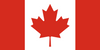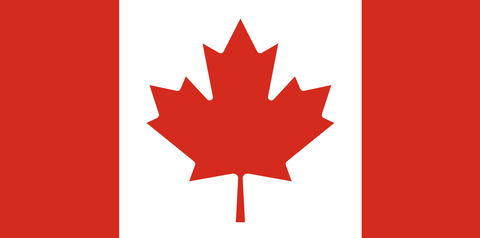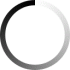Singapore Math® Essential Math is a series of two books and is written especially for the kindergarten student....
Workbooks provide further practice for students to complete independently in conjunction with textbook exercises. This essential component reinforces...
Singapore Math's Primary Math, US Edition series features the Concrete> Pictorial> Abstract approach. Students begin by learning through concrete and...
Workbooks provide further practice for students to complete independently in conjunction with textbook exercises. This essential component reinforces...
Singapore Math's Primary Math features the Concrete> Pictorial> Abstract approach. Students begin by learning through concrete and pictorial means...
Singapore Primary Math Workbooks are consumable and should be used in conjunction with the textbooks. Containing the exercises...
Singapore Math's Primary Math, U.S. Edition series features the Concrete> Pictorial> Abstract approach. Students begin by learning through concrete and...
Singapore Primary Math Workbooks are consumable and should be used in conjunction with the textbooks. Containing the exercises...
Singapore Math's Primary Math, U.S. Edition series features the Concrete> Pictorial> Abstract approach. Students begin by learning through concrete and...
Showing: 1-12 of 17